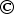Calculations based on 45" wide material, 44" after removing selvedge edges
 Squares Per Yard  ( 42" x 36")
 1" = 1584 5" = 56 9" = 16 1-1/2" = 696 5-1/2" = 48 9-1/2" = 12 1-7/8" = 437 5-7/8" = 42 9-7/8" = 12 2" = 396 6" = 42 10" = 12 2-1/2" = 238 6-1/2" = 30 10-1/2" = 12 2-7/8" = 180 6-7/8" = 30 10-7/8" = 12 3" = 168 7" = 35 11" = 12 3-1/2" = 120 7-1/2" = 20 11-1/2" = 9 3-7/8" = 99 7-7/8" = 20 11-7/8" = 9 4" = 99 8" = 20 12" = 9 4-1/2" = 72 8-1/2" = 20 12-1/2" = 6 4-7/8" = 63 8-7/8" = 16 12-7/8" = 6
 Squares Per Fat Quarter ( 18" x 22") 2" = 99 4" = 20 6" = 9 2-1/2" = 56 2-7/8" = 42 4-1/2" = 16 4-7/8" = 12 6-1/2" = 6 6-7/8" = 6 3" = 42 5" = 12 3-1/2" = 30 3-7/8" = 20 5-1/2" = 12 5-7/8" = 9
 Diagonal Block Measurements  To figure any other finished diagonal measurements just multiply the block size by x 1.41
 Finished Block Size Diagonal Measurement Decimal Equivalent 4" 5-5/8" 5.625" 5" 7-1/8" 7.125" 6" 8-1/2" 8.5 7" 9-7/8" 9.875 8" 11 3/8" 11.375" 9" 12 3/4" 12.75" 10" 14 1/8" 14.125" 12" 17" 17.0" 14" 19-3/4" 19.75"June 17 2002  Marcia Hohn